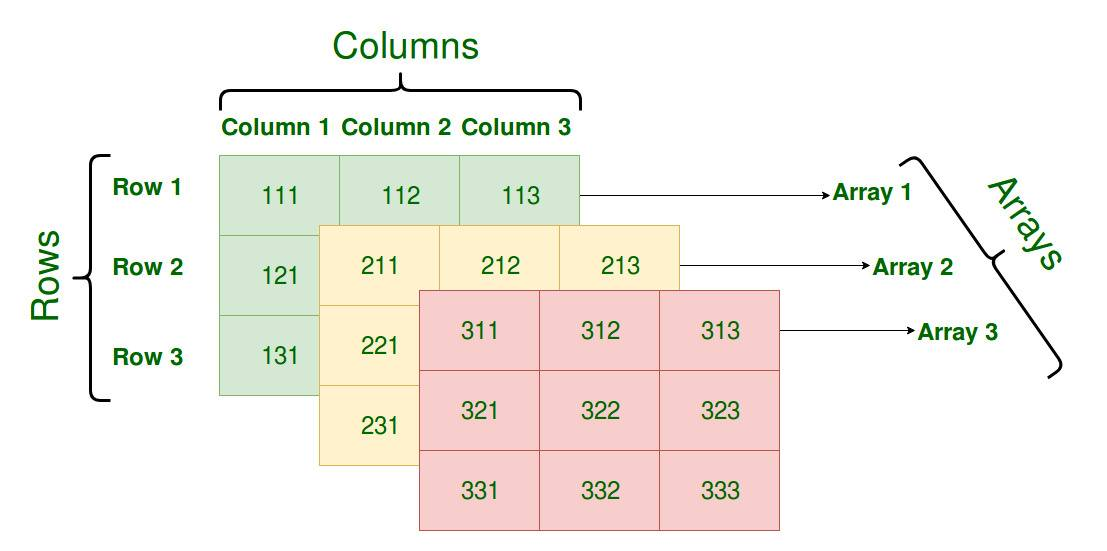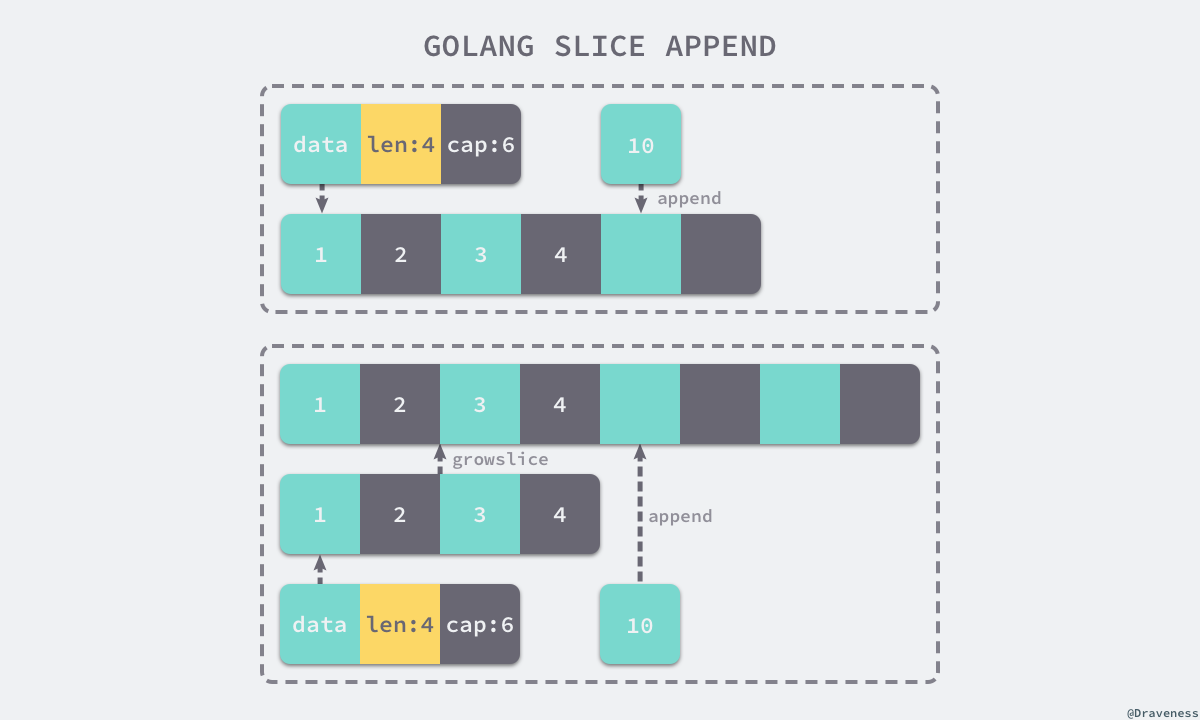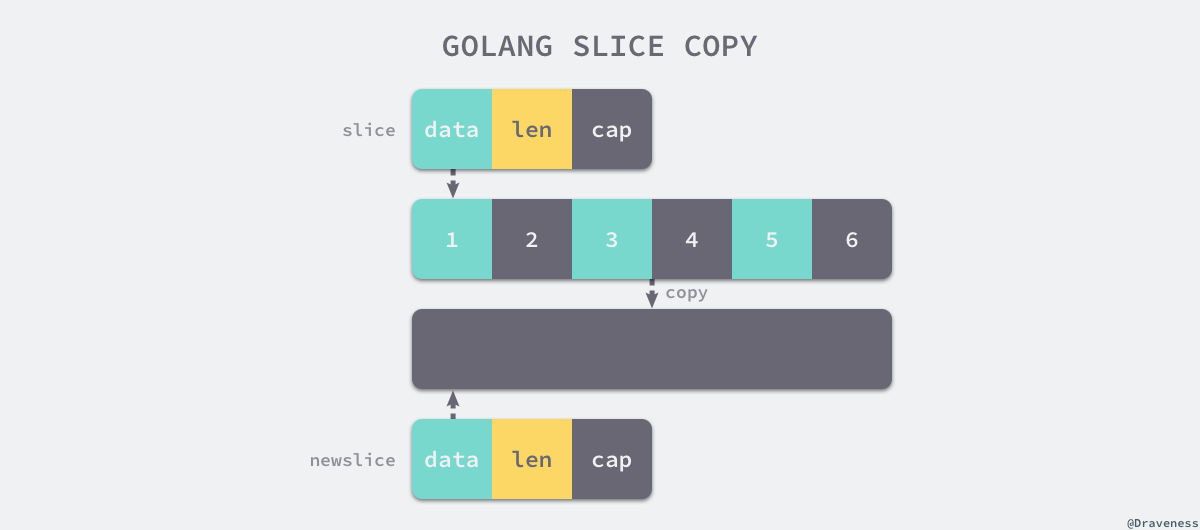# Go 语言数组和切片的原理# 3.1 数组和切片

## __ 1. 数组intinterface{}

Go 语言中数组的大小在初始化之后就无法改变，数组存储元素的类型相同，但是大小不同的数组类型在 Go 语言看来也是完全不同的，只有两个条件都相同才是同一个类型。

func NewArray(elem *Type, bound int64) *Type {    if bound < 0 {        Fatalf("NewArray: invalid bound %v", bound)    }    t := New(TARRAY)    t.Extra = &Array{Elem: elem, Bound: bound}    t.SetNotInHeap(elem.NotInHeap())    return t}

### __ 1.1. 创建

Go 语言中的数组有两种不同的创建方式，一种是我们显式指定数组的大小，另一种是编译器通过源代码自行推断数组的大小：

arr1 := int{1, 2, 3}arr2 := [...]int{1, 2, 3}

#### __ 上限推导

func typecheckcomplit(n *Node) (res *Node) {    // ...     switch t.Etype {    case TARRAY, TSLICE:        var length, i int64        nl := n.List.Slice()        for i2, l := range nl {            i++            if i > length {                length = i            }        }         if t.IsDDDArray() {            t.SetNumElem(length)        }    }}

#### __ 语句转换

func anylit(n *Node, var_ *Node, init *Nodes) {    t := n.Type    switch n.Op {    case OSTRUCTLIT, OARRAYLIT:        if n.List.Len() > 4 {            vstat := staticname(t)            vstat.Name.SetReadonly(true)             fixedlit(inNonInitFunction, initKindStatic, n, vstat, init)             a := nod(OAS, var_, vstat)            a = typecheck(a, ctxStmt)            a = walkexpr(a, init)            init.Append(a)            break        }         fixedlit(inInitFunction, initKindLocalCode, n, var_, init)    // ...    }}
1. 当元素数量小于或者等于 4 个时，会直接将数组中的元素放置在栈上；
2. 当元素数量大于 4 个时，会将数组中的元素放置到静态区并在运行时取出；

func fixedlit(ctxt initContext, kind initKind, n *Node, var_ *Node, init *Nodes) {    var splitnode func(*Node) (a *Node, value *Node)    // ...     for _, r := range n.List.Slice() {        a, value := splitnode(r)         a = nod(OAS, a, value)        a = typecheck(a, ctxStmt)        switch kind {        case initKindStatic:            genAsStatic(a)        case initKindLocalCode:            a = orderStmtInPlace(a, map[string][]*Node{})            a = walkstmt(a)            init.Append(a)        default:            Fatalf("fixedlit: bad kind %d", kind)        }    }}

var arr intarr = 1arr = 2arr = 3

func fixedlit(ctxt initContext, kind initKind, n *Node, var_ *Node, init *Nodes) {    var splitnode func(*Node) (a *Node, value *Node)    // ...     for _, r := range n.List.Slice() {        a, value := splitnode(r)         setlineno(value)        a = nod(OAS, a, value)        a = typecheck(a, ctxStmt)        switch kind {        case initKindStatic:            genAsStatic(a)        default:            Fatalf("fixedlit: bad kind %d", kind)        }     }}

var arr intstatictmp_0 = 1statictmp_0 = 2statictmp_0 = 3statictmp_0 = 4statictmp_0 = 5arr = statictmp_0

### __ 1.2. 访问和赋值func typecheck1(n *Node, top int) (res *Node) {    switch n.Op {    case OINDEX:        ok |= ctxExpr        l := n.Left        r := n.Right        t := l.Type        switch t.Etype {        case TSTRING, TARRAY, TSLICE:            why := "string"            if t.IsArray() {                why = "array"            } else if t.IsSlice() {                why = "slice"            }             if n.Right.Type != nil && !n.Right.Type.IsInteger() {                yyerror("non-integer %s index %v", why, n.Right)                break            }             if !n.Bounded() && Isconst(n.Right, CTINT) {                x := n.Right.Int64()                if x < 0 {                    yyerror("invalid %s index %v (index must be non-negative)", why, n.Right)                } else if t.IsArray() && x >= t.NumElem() {                    yyerror("invalid array index %v (out of bounds for %d-element array)", n.Right, t.NumElem())                } else if Isconst(n.Left, CTSTR) && x >= int64(len(n.Left.Val().U.(string))) {                    yyerror("invalid string index %v (out of bounds for %d-byte string)", n.Right, len(n.Left.Val().U.(string)))                }            }        }    //...    }}

PtrIndex <t> ptr idxLoad <t> ptr mem

LocalAddr {sym} base _PtrIndex <t> ptr idxStore {t} ptr val mem

## __ 2. 切片

[]int[]interface{}

func NewSlice(elem *Type) *Type {    if t := elem.Cache.slice; t != nil {        if t.Elem() != elem {            Fatalf("elem mismatch")        }        return t    }     t := New(TSLICE)    t.Extra = Slice{Elem: elem}    elem.Cache.slice = t    return t}

### __ 2.1. 结构

type SliceHeader struct {    Data uintptr    Len  int    Cap  int}

Data 作为一个指针指向的数组其实就是一片连续的内存空间，这片内存空间可以用于存储切片中保存的全部元素，数组其实就是一片连续的内存空间，数组中的元素只是逻辑上的概念，底层存储其实都是连续的，所以我们可以将切片理解成一片连续的内存空间加上长度与容量标识。### __ 2.2. 初始化

slice := []int{1, 2, 3}slice := make([]int, 10)

#### __ 字面量

var vstat intvstat = 1vstat = 2vstat = 3var vauto *int = new(int)*vauto = vstatslice := vauto[:]
1. 根据切片中的元素数量对底层数组的大小进行推断并创建一个数组；
2. 将这些字面量元素存储到初始化的数组中；
3. 创建一个同样指向 int 类型的数组指针；
4. 将静态存储区的数组 vstat 赋值给 vauto 指针所在的地址；
5. 通过 [:] 操作获取一个底层使用 vauto 的切片；

[:] 以及类似的操作 [:10] 其实都会在 SSA 代码生成 阶段被转换成 OpSliceMake 操作，这个操作会接受四个参数创建一个新的切片，切片元素类型、数组指针、切片大小和容量。

#### __ 关键字

func typecheck1(n *Node, top int) (res *Node) {    switch n.Op {    // ...    case OMAKE:        args := n.List.Slice()         i := 1        switch t.Etype {        case TSLICE:            if i >= len(args) {                yyerror("missing len argument to make(%v)", t)                return n            }             l = args[i]            i++            var r *Node            if i < len(args) {                r = args[i]            }             // ...            if Isconst(l, CTINT) && r != nil && Isconst(r, CTINT) && l.Val().U.(*Mpint).Cmp(r.Val().U.(*Mpint)) > 0 {                yyerror("len larger than cap in make(%v)", t)                return n            }             n.Left = l            n.Right = r            n.Op = OMAKESLICE        }    // ...    }}

make 参数的检查都是在 typecheck1 函数中完成的，它不仅会检查 len，而且会保证传入的容量 cap 一定大于或者等于 len；随后的中间代码生成阶段会把这里的 OMAKESLICE 类型的操作都转换成如下所示的函数调用：

makeslice(type, len, cap)

var arr intn := arr[:3]

func makeslice(et *_type, len, cap int) unsafe.Pointer {    mem, overflow := math.MulUintptr(et.size, uintptr(cap))    if overflow || mem > maxAlloc || len < 0 || len > cap {        mem, overflow := math.MulUintptr(et.size, uintptr(len))        if overflow || mem > maxAlloc || len < 0 {            panicmakeslicelen()        }        panicmakeslicecap()    }     return mallocgc(mem, et, true)}

func typecheck1(n *Node, top int) (res *Node) {    switch n.Op {    // ...    case OSLICEHEADER:    switch         t := n.Type        n.Left = typecheck(n.Left, ctxExpr)        l := typecheck(n.List.First(), ctxExpr)        c := typecheck(n.List.Second(), ctxExpr)        l = defaultlit(l, types.Types[TINT])        c = defaultlit(c, types.Types[TINT])         n.List.SetFirst(l)        n.List.SetSecond(c)    // ...    }}

OSLICEHEADER 操作会创建一个如下所示的结构体，其中包含数组指针、切片长度和容量，它是切片在运行时的表示：

type SliceHeader struct {    Data uintptr    Len  int    Cap  int}

### __ 2.3. 访问

func (s *state) expr(n *Node) *ssa.Value {    switch n.Op {    case OLEN, OCAP:        switch {        case n.Left.Type.IsSlice():            op := ssa.OpSliceLen            if n.Op == OCAP {                op = ssa.OpSliceCap            }            return s.newValue1(op, types.Types[TINT], s.expr(n.Left))        // ...        }    // ...    }}

func (s *state) expr(n *Node) *ssa.Value {    switch n.Op {    case OINDEX:        switch {        case n.Left.Type.IsSlice():            p := s.addr(n, false)            return s.load(n.Left.Type.Elem(), p)        // ...        }    // ...    }}

### __ 2.4. 追加

ptr, len, cap := slicenewlen := len + 3if newlen > cap {    ptr, len, cap = growslice(slice, newlen)    newlen = len + 3}*(ptr+len) = 1*(ptr+len+1) = 2*(ptr+len+2) = 3return makeslice(ptr, newlen, cap)

a := &sliceptr, len, cap := slicenewlen := len + 3if uint(newlen) > uint(cap) {   newptr, len, newcap = growslice(slice, newlen)   vardef(a)   *a.cap = newcap   *a.ptr = newptr}newlen = len + 3*a.len = newlen*(ptr+len) = 1*(ptr+len+1) = 2*(ptr+len+2) = 3func growslice(et *_type, old slice, cap int) slice {    newcap := old.cap    doublecap := newcap + newcap    if cap > doublecap {        newcap = cap    } else {        if old.len < 1024 {            newcap = doublecap        } else {            for 0 < newcap && newcap < cap {                newcap += newcap / 4            }            if newcap <= 0 {                newcap = cap            }        }    }

1. 如果期望容量大于当前容量的两倍就会使用期望容量；
2. 如果当前切片容量小于 1024 就会将容量翻倍；
3. 如果当前切片容量大于 1024 就会每次增加 25% 的容量，直到新容量大于期望容量；

var overflow bool    var lenmem, newlenmem, capmem uintptr    switch {    // ...    default:        lenmem = uintptr(old.len) * et.size        newlenmem = uintptr(cap) * et.size        capmem, overflow = math.MulUintptr(et.size, uintptr(newcap))        capmem = roundupsize(capmem)        newcap = int(capmem / et.size)    }     var p unsafe.Pointer    if et.kind&kindNoPointers != 0 {        p = mallocgc(capmem, nil, false)        memclrNoHeapPointers(add(p, newlenmem), capmem-newlenmem)    } else {        p = mallocgc(capmem, et, true)        if writeBarrier.enabled {            bulkBarrierPreWriteSrcOnly(uintptr(p), uintptr(old.array), lenmem)        }    }    memmove(p, old.array, lenmem)     return slice{p, old.len, newcap}}

TEXT runtime·memclrNoHeapPointers(SB), NOSPLIT, $0-16 MOVD ptr+0(FP), R0 MOVD n+8(FP), R1 loop: Loop Get R1 I64Eqz If RET End Get R0 I32WrapI64 I64Const$0        I64Store8 $0 Get R0 I64Const$1        I64Add        Set R0         Get R1        I64Const \$1        I64Sub        Set R1         Br loop    End    UNDEF

growslice 函数最终会返回一个新的 slice 结构，其中包含了新的数组指针、大小和容量，这个返回的三元组最终会改变原有的切片，帮助 append 完成元素追加的功能。

### __ 2.5. 拷贝

func slicecopy(to, fm slice, width uintptr) int {    if fm.len == 0 || to.len == 0 {        return 0    }     n := fm.len    if to.len < n {        n = to.len    }     if width == 0 {        return n    }     // ...     size := uintptr(n) * width    if size == 1 {        *(*byte)(to.array) = *(*byte)(fm.array)    } else {        memmove(to.array, fm.array, size)    }    return n}## __ 5. 其他

### __ 5.1. 关于图片和转载

** 本文转载自 Draveness 技术博客。